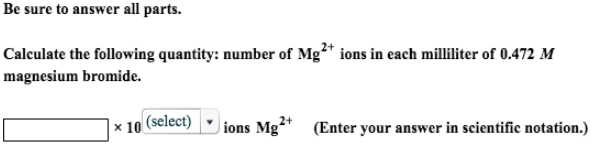# Problem: Be sure to answer all parts.Calculate the following quantity: number of Mg 2+ ions in each milliliter of 0.472 M magnesium bromide.

###### FREE Expert Solution
83% (75 ratings)###### Problem Details

Be sure to answer all parts.

Calculate the following quantity: number of Mg 2+ ions in each milliliter of 0.472 M magnesium bromide.